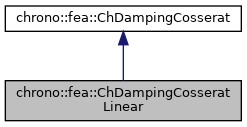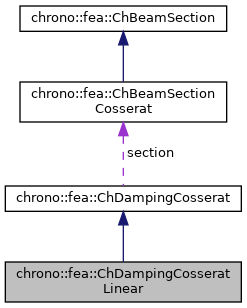chrono::fea::ChDampingCosseratLinear Class Reference

## Description

Simple linear lumped damping of beam sections of Cosserat type, {n,m}=f({e',k'}) where damping is proportional to speed of deformation/curvature via linear constants:

  n = R_e * e'
m = R_k * k'


#include <ChBeamSectionCosserat.h>

Inheritance diagram for chrono::fea::ChDampingCosseratLinear:[legend]
Collaboration diagram for chrono::fea::ChDampingCosseratLinear:[legend]

## Public Member Functions

virtual void ComputeStress (ChVector<> &stress_n, ChVector<> &stress_m, const ChVector<> &dstrain_e, const ChVector<> &dstrain_k) override
Compute the generalized cut force and cut torque, caused by structural damping, given actual deformation speed and curvature speed. More...

virtual void ComputeDampingMatrix (ChMatrixNM< double, 6, 6 > &R, const ChVector<> &dstrain_e, const ChVector<> &dstrain_k) override
Compute the 6x6 tangent material damping matrix, ie the jacobian [Rm]=dstress/dstrainspeed. More...

ChVector GetDampingCoefficientsRe ()

void SetDampingCoefficientsRe (const ChVector<> mR_e)

ChVector GetDampingCoefficientsRk ()

void SetDampingCoefficientsRk (const ChVector<> mR_k)Public Attributes inherited from chrono::fea::ChDampingCosserat
ChBeamSectionCosseratsection

## ◆ ComputeDampingMatrix()

 void chrono::fea::ChDampingCosseratLinear::ComputeDampingMatrix ( ChMatrixNM< double, 6, 6 > & R, const ChVector<> & dstrain_e, const ChVector<> & dstrain_k )
overridevirtual

Compute the 6x6 tangent material damping matrix, ie the jacobian [Rm]=dstress/dstrainspeed.

By the way, in this model, it is simply a diagonal matrix with R_e and R_k values on the diagonal.

Parameters
 R 6x6 material stiffness matrix values here dstrain_e current strain speed (deformation part) dstrain_k current strain speed (curvature part)

Reimplemented from chrono::fea::ChDampingCosserat.

## ◆ ComputeStress()

 void chrono::fea::ChDampingCosseratLinear::ComputeStress ( ChVector<> & stress_n, ChVector<> & stress_m, const ChVector<> & dstrain_e, const ChVector<> & dstrain_k )
overridevirtual

Compute the generalized cut force and cut torque, caused by structural damping, given actual deformation speed and curvature speed.

Parameters
 stress_n local stress (generalized force), x component = traction along beam stress_m local stress (generalized torque), x component = torsion torque along beam dstrain_e local strain speed (deformation); x elongation speed; y,z shear speeds dstrain_k local strain speed (curvature); x torsion speed; y,z line curvature speeds

Implements chrono::fea::ChDampingCosserat.

The documentation for this class was generated from the following files:
• /builds/uwsbel/chrono/src/chrono/fea/ChBeamSectionCosserat.h
• /builds/uwsbel/chrono/src/chrono/fea/ChBeamSectionCosserat.cpp